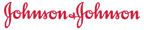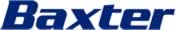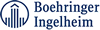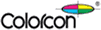➤ Get the DrugPatentWatch Daily Briefing

Get Daily Updates on Generic Entry, Litigation, Biosimilars, and more …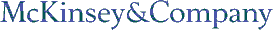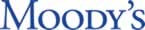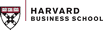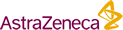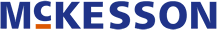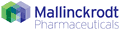Last Updated: September 22, 2021

DrugPatentWatch Database Preview

# Claims for Patent: 5,808,146

« Back to Dashboard

##### Summary for Patent: 5,808,146
 Title: Amino acid analogs for tumor imaging Abstract: The invention provides novel amino acid compounds of use in detecting and evaluating brain and body tumors. These compounds combine the advantageous properties of 1-amino-cycloalkyl-1-carboxylic acids, namely, their rapid uptake and prolonged retention in tumors with the properties of halogen substituents, including certain useful halogen isotopes including fluorine-18, iodine-123, iodine-125, iodine-131, bromine-75, bromine-76, bromine-77 and bromine-82. In one aspect, the invention features amino acid compounds that have a high specificity for target sites when administered to a subject in vivo. Preferred amino acid compounds show a target to non-target ratio of at least 5:1, are stable in vivo and substantially localized to target within 1 hour after administration. An especially preferred amino acid compound is [.sup.18 F]-1-amino-3-fluorocyclobutane-1-carboxylic acid (FACBC). In another aspect, the invention features pharmaceutical compositions comprised of an .alpha.-amino acid moiety attached to either a four, five, or a six member carbon-chain ring. In addition, the invention features analogs of .alpha.-aminoisobutyric acid. Inventor(s): Goodman; Mark M. (Atlanta, GA), Shoup; Timothy (Decatur, GA) Assignee: Emory University (Atlanta, GA) Application Number: 08/554,906 Patent Claims: 1. An amino acid analog having the general structure ##STR20## where R.sub.1 is X, X--CH.dbd.CH--, or R.sub.3 R.sub.2 is H, or R.sub.3 if R.sub.1 is R.sub.3, ##STR21## such that ##STR22## is formed where x is 0 or 1, y is 1 or 2, z is 1, 2, 3 or 4 and z>y if y is 2, q is 1 or 0 if n is 1 and j is 0, n is 1 or 2, but 0 if m is 0, m is 0 or 1, j is 0 or 1, and X is .sup.18 F, .sup.123 I, .sup.125 I, .sup.131 I, .sup.75 Br, .sup.76 Br, .sup.77 Br, or .sup.82 Br. 2. A compound of claim 1, wherein R.sub.1 and R.sub.2 =R.sub.3. 3. A cyclic compound according to claim 1 wherein x is 0 y is 1 z is 2 q is 1 m is 0, and j is 0. 4. A compound according to claim 3 wherein X is .sup.18 F, or .sup.123 I. 5. A compound according to claim 3 wherein X is .sup.18 F. 6. A compound according to claim 1 wherein R.sub.1 and R.sub.2 =R.sub.3, x is 0 or 1 y is 2 z is 4 q is 1 m and j are each 0, and X is .sup.18 F, or .sup.123 I. 7. A compound according to claim 6 wherein x is 1 X is .sup.18 F. 8. The compound of claim 8 wherein x is 0 and X is .sup.123 I. 9. A compound according to claim 6 wherein x is 1 and X is .sup.123 I. 10. A compound according to claim 1 wherein R.sub.1 and R.sub.2 =R.sub.3 x is 0 y is 1 z is 2 q is 0 m is 1 n is 1 j is 0, and X is .sup.18 F, or .sup.123 I. 11. A compound according to claim 10 wherein X is .sup.18 F. 12. A compound according to claim 1 wherein R.sub.1 and R.sub.2 =R.sub.3 x is 1 y is 1 z is 1 q is 0 m and j are 0, and X is .sup.18 F, or .sup.123 I. 13. A compound according to claim 12 wherein X is .sup.123 I. 14. A compound according to claim 1 wherein R.sub.1 and R.sub.2 =R.sub.3 x is 0 y is 1 z is 2 q is 1 m is 1 n is 1 j is 1, and X is .sup.18 F, or .sup.123 I. 15. The compound of claim 14 wherein X is .sup.123 I. 16. A compound according to claim 1 wherein R.sub.1 and R.sub.2 =R.sub.3 x is 0 y is 1 z is 2 q is 0 m is 0 j is 1, and X is .sup.18 F, or .sup.123 I. 17. The compound of claim 16 wherein X is .sup.123 I. 18. A compound according to claim 1 wherein R.sub.1 and R.sub.2 =R.sub.3 x is 0 or 1 y is 2 z is 4 q is 1 m is 1 n is 1 j is 1, and X is .sup.18 F, or .sup.123 I. 19. The compound of claim 18 wherein X is .sup.18 F. 20. The compound of claim 18 wherein X is .sup.123 I. 21. A compound according to claim 1 wherein R.sub.1 and R.sub.2 =R.sub.3 x is 0 or 1 y is 2 z is 4 q is 0 m is 0 j is 1, and X is .sup.18 F, or .sup.123 I. 22. The compound of claim 21 wherein X is .sup.18 F. 23. The compound of claim 21 wherein X is .sup.123 I. 24. A compound of claim 1 wherein R.sub.1 and R.sub.2 .noteq.R.sub.3. 25. A compound according to claim 24 wherein X is .sup.18 F. 26. A compound according to claim 1 wherein R.sub.1 is X--CH.dbd.CH--, R.sub.2 is H, y is 1 and z is 2. 27. A compound of claim 26 wherein X is .sup.123 I.

### Make Better Decisions: Try a trial or see plans & pricing